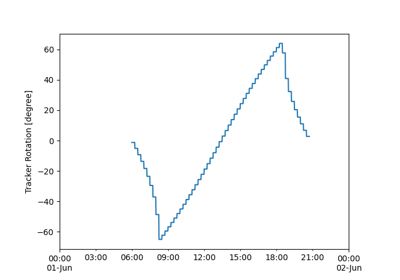pvlib.pvsystem.SingleAxisTrackerMount¶

class pvlib.pvsystem.SingleAxisTrackerMount(axis_tilt: float = 0.0, axis_azimuth: float = 0.0, max_angle: float = 90.0, backtrack: bool = True, gcr: float = 0.2857142857142857, cross_axis_tilt: float = 0.0, racking_model: Optional[str] = None, module_height: Optional[float] = None)[source]

Single-axis tracker racking for dynamic solar tracking.

Parameters
• axis_tilt (float, default 0) – The tilt of the axis of rotation (i.e, the y-axis defined by axis_azimuth) with respect to horizontal. [degrees]

• axis_azimuth (float, default 180) – A value denoting the compass direction along which the axis of rotation lies, measured east of north. [degrees]

• max_angle (float, default 90) – A value denoting the maximum rotation angle of the one-axis tracker from its horizontal position (horizontal if axis_tilt = 0). A max_angle of 90 degrees allows the tracker to rotate to a vertical position to point the panel towards a horizon. max_angle of 180 degrees allows for full rotation. [degrees]

• backtrack (bool, default True) – Controls whether the tracker has the capability to “backtrack” to avoid row-to-row shading. False denotes no backtrack capability. True denotes backtrack capability.

• gcr (float, default 2.0/7.0) – A value denoting the ground coverage ratio of a tracker system which utilizes backtracking; i.e. the ratio between the PV array surface area to total ground area. A tracker system with modules 2 meters wide, centered on the tracking axis, with 6 meters between the tracking axes has a gcr of 2/6=0.333. If gcr is not provided, a gcr of 2/7 is default. gcr must be <=1. [unitless]

• cross_axis_tilt (float, default 0.0) – The angle, relative to horizontal, of the line formed by the intersection between the slope containing the tracker axes and a plane perpendicular to the tracker axes. Cross-axis tilt should be specified using a right-handed convention. For example, trackers with axis azimuth of 180 degrees (heading south) will have a negative cross-axis tilt if the tracker axes plane slopes down to the east and positive cross-axis tilt if the tracker axes plane slopes up to the east. Use calc_cross_axis_tilt() to calculate cross_axis_tilt. [degrees]

• racking_model (str, optional) – Valid strings are ‘open_rack’, ‘close_mount’, and ‘insulated_back’. Used to identify a parameter set for the SAPM cell temperature model.

• module_height (float, optional) – The height above ground of the center of the module [m]. Used for the Fuentes cell temperature model.

Methods

 __init__([axis_tilt, axis_azimuth, …]) Initialize self. get_orientation(solar_zenith, solar_azimuth) Determine module orientation.

Attributes

 axis_azimuth axis_tilt backtrack cross_axis_tilt gcr max_angle module_height racking_model

Examples using pvlib.pvsystem.SingleAxisTrackerMount¶